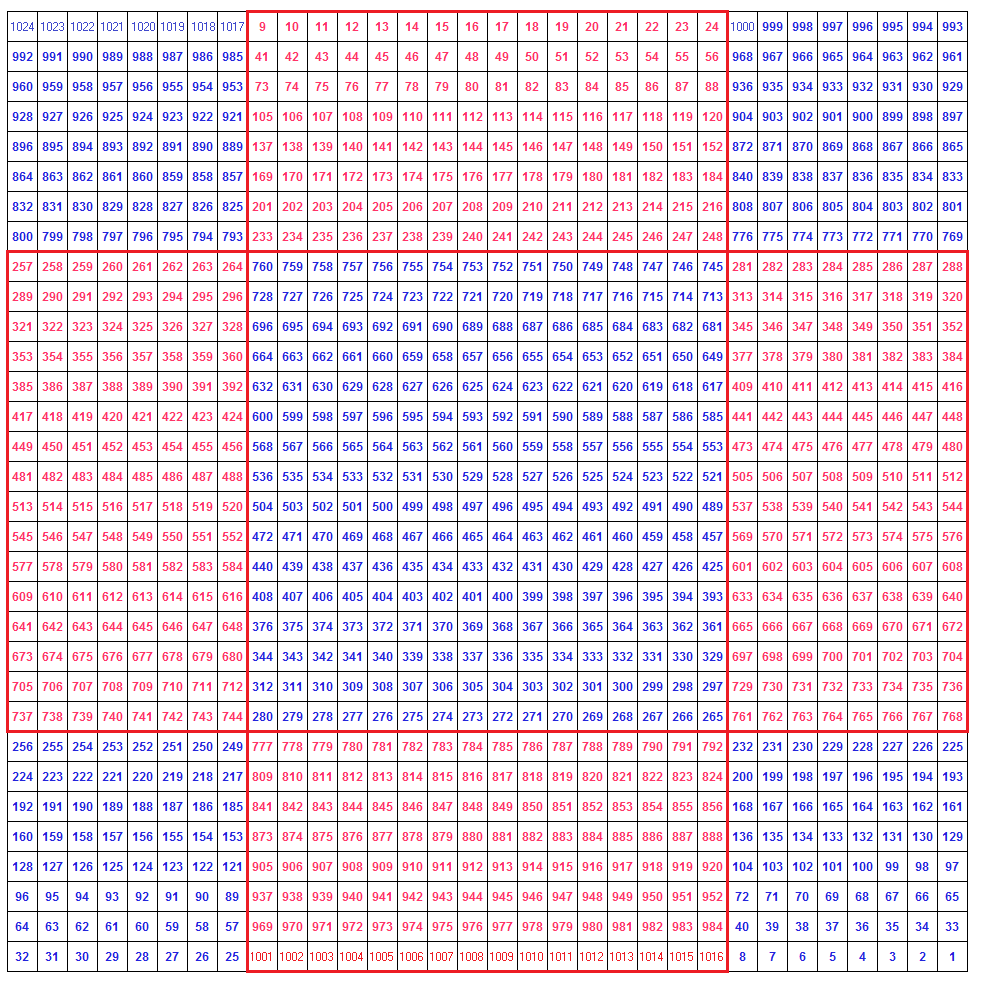Links to Other Magic Square Pages:
Odd Magic Squares
Singly Even Magic Squares

# Even Magic Squares (Part 2)

#### Doubly Even Magic Squares

The term "doubly even" isn't very descriptive and all it means is a multiple of four. So, now we'll discuss the construction of magic squares where n=4, 8, 12 and so on.

A 4 by 4 magic square is a doubly even magic square, one of the three types of magic square.
The other two types are:
odd (where n=3, 5, 7, 9, 11, etc.)
singly even (even but not a multiple of 4 where n=6, 10, 14, 18, 22, etc.)

Now, let's discuss the construction of doubly even magic squares, starting with the simplest, the 4 row by 4 column one.
To determine the sum of any normal magic square we use the formula: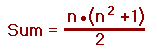So, for the 4 by 4 magic square, each row, each column and both diagonals would sum to
4 • (4² + 1) ÷ 2 = 34

Here is a simple, easy to remember way to make a 4 by 4 magic square.
In a 4 by 4 grid write the numbers 1 through 16 from left to right.
Now "flip" the numbers in the diagonals (the red lines). That is to say, exchange 16 & 1, 6 & 11, 13 & 4 and 10 & 7, and you'll have a magic square totalling 34.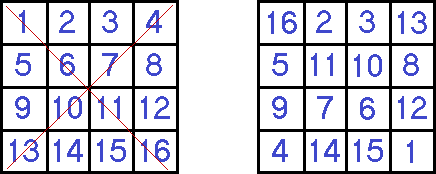Unfortunately, the above method only works for the 4 by 4 square and so we'll have to learn another way for constructing doubly even magic squares of any size.

Looking at the diagram below, divide the square into four "mini-squares" - squares at the four corners, the size of each equals n/4.
A 4 by 4 magic square has mini-squares that are 1 by 1. (note the 4 red squares).
For an 8 by 8 the mini-squares are 2 by 2, for a 12 by 12 the mini-squares are 3 by 3 and so on.

Next, divide the center into a large square the size of which is n/2.
For a 4 by 4 square the size would be 2. (note the blue square)
Now, we fill in the square with the numbers from 1 through 16 but only for the squares that have an 'M' or 'L' in it and we leave the others blank.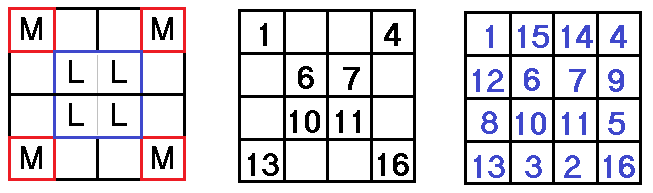Finally, starting at the top left cell, counting backwards from 16, only fill in the blank cells and then the square is completed. (see the square at the right)
This method works perfectly but gets a bit confusing with the "mini-squares", the large square and the formula for each.

Let's try building an 8 by 8 square with a different approach. For this method, we will divide up the square into smaller squares, each of which has a side equal to n/4. We will place these 2 by 2 squares along both diagonals of the square. (see illustration)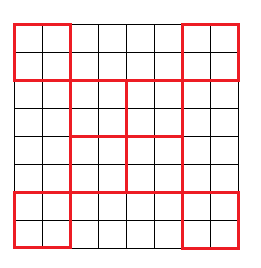Counting from 1 to 64, starting from the top left and only filling in numbers that fall within the red squares (while leaving the others blank) produces this partially completed magic square: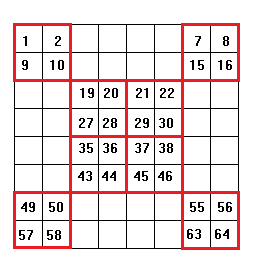Starting at the top leftmost cell, while counting from 64 then backwards to 1, insert this number whenever a blank cell is encountered. For example, we can't fill in 64 or 63 because 1 and 2 are in those cells. However, when we reach 62, 61, 60 and 59 we can fill in those numbers because those squares are blank.
Then we continue without filling in 58, 57, 56 and 55 but 54, 53, 52 and 51 are blank cells and do get filled in.
Continuing in this way, until the bottom right cell is encountered, the magic square is now complete with each row, column and diagonal summing to 260.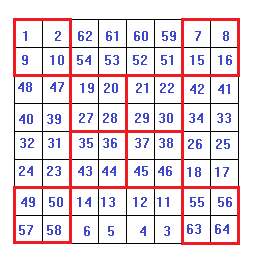##### A Third Solution

This is similar to the previous two solutions but is much easier to understand and to memorize.
This time the n=8 square is divided into rectangles, each of which has a dimension of n/2 by n/4. The red lines indicate where to place the numbers 1 through 64 if the number is within a rectangle.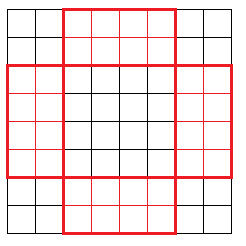Following these rules, the partially-completed magic square will look like this: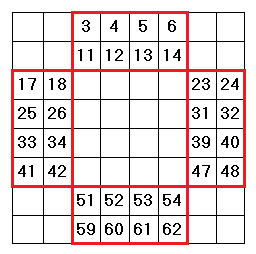Starting from the top left and counting backwards from 64 to 1, fill in the blank cells of the square and you have the finished square.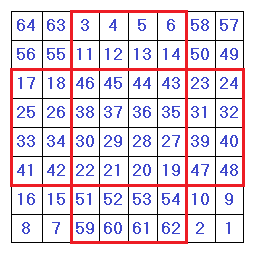The 32 by 32 magic square shown below was also created by using the "rectangle method". The numbers in red are the ones that are in sequential order and the blue numbers are the reverse order numbers. The "magic sum" of this square is 16,400.